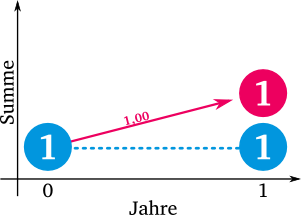# How we compute e 2

## The number e

Next to π is the Euler's numbere the best known constant in mathematics. It is particularly common in calculus, as it is the only known function where the output function, derivative and integral are identical. It is difficult to portray because it turns out to be irrational number can not be written as a fraction. Nor can it be represented as the solution of a polynomial equation, which makes it a tranquil number makes. Hence it is necessary to find another way to represent e.

### Why is e so important?

Before we get into the derivation of the number e, let's clarify why the number e is so important in mathematics. On the one hand, e is the only function where the derivative, antiderivative, and output function are identical. The value of e is found in many mathematical formulas, such as describing an exponential increase or decrease in growth or decay (including compound interest), the bell curve in statistics, the shape of a hanging cable or a standing bow. e can also be seen in some problems of probability and the distribution of prime numbers.

In the field of materials testing in engineering, the number e is used extensively in formulas such as those used in the formulas for ultrasonic damping. The sound energy decays by a factor with increasing distance from the sound source, based on e. Because the number e occurs with a certain frequency in the world around us, it is also used as the base of the natural logarithm. Trigonometric and hyperbolic functions can also be written as exponential functions.

### Derivation with explanationLet's say we deposit \$ 1 into an account in the bank that has an interest rate of 100%. The formula for calculating interest and compound interest for a start-up capital of K0 with an interest rate of p for a period of n:For the investment of a single euro this would be:Let's assume that our bank grants us very generous terms. Our money doubles in a year. So we get an interest rate p of 100%. After one year, we therefore have € 2. But as you can see from the graph, we receive all of our interest on the last day of the year. That does not correspond to the reality. Let's take a closer look and divide the year (12 months) by two. We now get two times six months. In this case we will receive 50% interest every six months. This corresponds to the formulaWe not only receive interest on our original euro, but also on the € 0.50 that accrued after six months. The arrows in the graphic indicate how the money was made:

2. After six months, our euro has already earned € 0.50 interest. A red arrow goes from the starting euro to € 0.50 for six months. At the same time, the original € 0.50 is retained (horizontal arrows). The sum of both amounts is € 1.00 (in red, after 12 months).
3. After a year, a lot has happened: our starter euro has earned € 0.50 for us again. But the € 0.50 from before also earned € 0.25. Together we have € 2.25.

With two interest rates of 50% each, we now have € 2.25 in our account after one year. With that we earned € 1.25. At € 0.25, that's a little more than we had with a single interest rate of 100%.But what happens if we increase the interest intervals even further? We now divide 12 months by three and now get 33% every four months (to be precise:).

We start again with one euro. Thanks to three payments and the compound interest effect, we had just under € 2.37 together at the end of the year. So we earned € 1.37. Again, that's more than just two payouts. However, the difference is no longer as great as it was before, when we expected two payments per year.

What happens if we let the money be paid out to us at ever smaller intervals? Can we earn infinitely much by dividing the 100% infinitely and getting paid out infinitely?
No, unfortunately not. There is a limit, and it is precisely this limit that is e! Just like the speed of light is the cosmic speed limit, so there is maximum possible growth from 100%.

### determine e approximately

nvalue
102.5937424601
1002.704813829421526
1.0002.716923932235893
5.0002.718010050101854
10.0002.718145926825225
20.0002.718213874527883
50.0002,718254646139102
100.0002,718268237174489
1.000.0002,718280469319376
10.000.0002,718281692544966
100.000.0002,718281814867636
1.000.000.0002,718281827099904
1.000.000.000.0002,718281828457686
1.000.000.000.000.0002,718281828459043

With the formulawe can look at what happens when the number of 100% interest payments keeps increasing. What happens if we pay interest on our euros every month, every day, every second or even more frequently?

The bigger n gets, the closer we get to e! With such large values ​​of n, it is astonishing that the Swiss mathematician Leonard Euler was the first to calculate the number e to 23 decimal places as early as 1748. e is therefore also defined mathematically with the help of the limit value, as follows:n is getting closer and closer to the infinite. The larger n becomes, the less the calculated value deviates from the actual value e. The table on the left shows how the formula behaves for large values ​​of n. The underlined numbers correspond to the actual value of e. Currently (2012) 1,000,000,000,000 decimal places of e are known.